# Abstract

The Equivalence Theorem is commonly used to calculate perturbatively amplitudes involving gauge bosons at energy scales higher than gauge boson masses. However, when the scalar sector is strongly interacting the theory is non-perturbative. We show that the Equivalence Theorem holds in the large N limit at next-to-leading order by calculating the decay widths h -> W+W- and h <FONT FACE=Symbol>® p</FONT>+pi-. We also show, in the same scheme of calculations, that unitarity is fulfilled for the process h <FONT FACE=Symbol>® p</FONT>+pi-.

Higgs boson decay in the large N limit

John MoralesI,II; R. MartinezI; Rafael HurtadoI,II; Rodolfo A. DiazI

IUniversidad Nacional de Colombia, Departamento de Física, Bogotá, Colombia

IICentro Internacional de Física, Bogotá, Colombia

ABSTRACT

The Equivalence Theorem is commonly used to calculate perturbatively amplitudes involving gauge bosons at energy scales higher than gauge boson masses. However, when the scalar sector is strongly interacting the theory is non-perturbative. We show that the Equivalence Theorem holds in the large N limit at next-to-leading order by calculating the decay widths h ® W+W- and h ® p+p-. We also show, in the same scheme of calculations, that unitarity is fulfilled for the process h ® p+p-.

I. INTRODUCTION

The Standard Model (SM) of the electroweak interactions, based on the SU(2)L Ä U(1)Y gauge symmetry , is a successful theory and agrees with most experimental results . However, the scalar sector responsible for the symmetry breaking of the SM is not well known and it has not been tested yet. This sector gives masses to the particles of the model, fermions and gauge fields, when the scalar field has a non vanishing Vacuum Expectation Value (VEV) after the symmetry breaking. In the scalar sector a Higgs particle appears with a mass given by= 2lv2, where l is the coupling constant of the self-interacting term and v is the VEV (v » 246 GeV). mh is an unknown parameter so far.

Nevertheless, the precision tests of the SM impose strong bounds to the Higgs mass when the scalar sector is weakly-coupled. The results from LEP Electroweak Working Group analysis yield mh =GeV (68% CL) , and an upper limit of mh < 260 GeV with one-sided 95% CL . The direct search of the Higgs boson done at LEP gives a lower limit of mh > 114.4 GeV . On the other hand, it is possible to have different models beyond the SM with a heavy Higgs with a mass lying in the TeV scale for a strongly interacting scalar sector. However, for this scenario to be held, the new physics contributions must cancel those ones introduced by the heavy Higgs particle at low energies .

If the SM is an effective theory derived from a more fundamental one, then there is an associated L scale for the appearance of new physics. The use of theoretical arguments, like unitarity , triviality  and vacuum stability , may allow to get constraints for these two parameters (L, mh) .

The upper limit for the Higgs mass can be obtained by triviality considerations in the Higgs sector . When the quartic coupling constant l in the scalar sector of the Higgs potential is renormalized introducing a cut-off L, the coupling goes to zero when L goes to infinity, implying that mh goes to zero. This is not the case for the SM, because it needs a massive scalar particle at low energies to explain experimental results, and then the SM can be considered as an effective theory below a given energy scale. If we knew this scale we could predict the Higgs mass. Further, if the SM had a Higgs with a mass around 1 TeV, then the scalar sector would be strongly interacting and the underlying theory would become non-perturbative.

The amplitude for a heavy Higgs decaying into two longitudinally polarized gauge bosons readsBy considering that in the perturbation expansion the l2 term must be smaller than the l term, it is found that l(mh) » 7 implying that mh » 1 TeV. On the other hand, using the scattering process WW ® ZZ mediated by a Higgs particle, which might be important in future collider experiments like LHC and linear colliders, the cross section for energies>> mh at two loops level is given byThis cross section is negative for some values of l which means that the perturbative expansion breaks down. Considering that the l2 term must be smaller than the l term, a necessary condition to have a convergent series is l » 4, in this case mh » 700 GeV . The above scenarios correspond to the limit between weakly-coupled and strongly-coupled scalar sectors.

In the Marciano and Willenbrock paper  they calculated the decays of a heavy Higgs boson up to O(g2/) in perturbation theory using the Equivalence Theorem (ET) , from which the amplitude with gauge bosons longitudinally polarized at energies O(q2 >>) is equivalent to the same amplitude but changing the corresponding longitudinal components by the would-be Goldstone bosons. For Higgs masses of the order of mh » 1 TeV and mh » 1.3 TeV the radiative corrections for the decay h ® W+W- are 7.3% and 12%, respectively. At this scale the scalar sector is strongly-coupled and the theory is non-perturbative. It is obvious that the amplitude at next-to-leading order breaks the perturbative expansion because all Feynman rules are proportional to the Higgs mass. For strongly interacting models is necessary to use a non-perturbative method to calculate radiative corrections and get bounded amplitudes. While it has been shown that the ET holds order by order in perturbation theory, it has not been confirmed that it does in non-perturbative calculations.

Due to the importance of studying the Higgs dynamics in non-perturbative regimes, a formalism was introduced in Ref.  which uses Chiral Perturbation Theory(cPT) . Amplitudes are obtained as a power expansion in the energy, this implies that the conventional ET does not hold anymore . Thus, a new formalism is necessary to have an effective theory .

The large N limit is an alternative approach that predicts bounded positive defined amplitudes, consistent with pion dispersion , and useful to study the symmetry breaking of the strongly interacting sector . The scalar sector of the SM can be modelled by a Linear Sigma Model O(4) and then generalized to a model with O(N + 1) symmetry. This method has been applied to study the Higgs boson at TeV energy scales [10, 22]. We show that the large N limit can predict amplitudes that fulfill the ET and the unitarity condition at next-to-leading order for the SM, with a strongly interacting scalar sector, by using the h ® W+W- and h ® p+p- processes.

In section 2 we introduce the Gauged Linear Sigma Model O(N + 1). In section 3 we calculate the Higgs decay widths, h ® W+W- and h ® p+p-, in the large N limit and we show that the ET holds at next-to-leading order. In section 4 we show that the amplitude h ® p+p- satisfies unitarity in the large N limit. In section 5 we give our conclusions.

II THE O(N + 1) MODEL

It is well known that the Linear Sigma Model represents the symmetry breaking O(N + 1) ® O(N) with N would-be Goldstone bosons which belong to the fundamental irreducible representation of the remaining symmetry O(N). For the purposes of this work the would-be Goldstone bosons will be named like pions p. For a gauge invariant model under SU(2)L Ä U(1)Y local symmetry the large N limit for the SM is defined as

Lg = LYM + (DmF)(DmF) - V(F2)

with F = (p1,p2,...,pN,s) and F2 = FF. As usual LYM is the Yang-Mills Lagrangian of the SM and the covariant derivative is defined as

where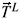= -(i/2)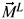are the generators of the SU(2)L gauge group and= -(i/2)MY is the generator of the U(1)Y 4gauge group. The M matrices are given by 

which belong to an irreducible representation of the O(N + 1) Lie algebra with i,j = 1,2,3 and a,b = 1,2,...,N + 1. The matrices which belong to the adjoint representation of the SU(2)L Lie algebra are given by

and, the corresponding matrix for the U(1)Y Lie algebra reads

where dots represent zeros. In this form we have a global O(N + 1) symmetry with a local SU(2)L Ä U(1)Y symmetry.

The Higgs potential, invariant under O(N + 1), can be written as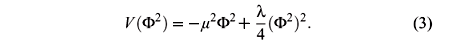Aligning the vacuum state as áfñ0º (0,...,v), with F2 = v2 = 2µ2/l, the global symmetry O(N + 1) is broken to O(N) and the local symmetry is broken as SU(2)L Ä U(1)Y ® U(1)Q. By defining the Higgs field as h = s - v, we find the following expressionThe gauge boson masses are obtained from the kinetic term,where the mass eigenstates are given byand qW is the Weinberg angle with tan qW = g'/g. Thefields, with mW = gv/2 » 80.6 GeV masses, are the charged gauge bosons, and the Zm field, with mZ = v(g2 + g'2)1/2/2 » 91.2 GeV mass, is the weak neutral gauge boson. The Am field is the massless photon.

The Lagrangian has terms of the form g2vmpa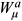/4, mixing gauge bosons with would-be Goldstone bosons, which can be cancelled by gauge fixing. We choose the Landau gauge (x = 0) because in this gauge a lot of Feynman diagrams cancel or suppress, the pa fields do not couple to the ghost fields, and their propagators are massless. The final Lagrangian can be written as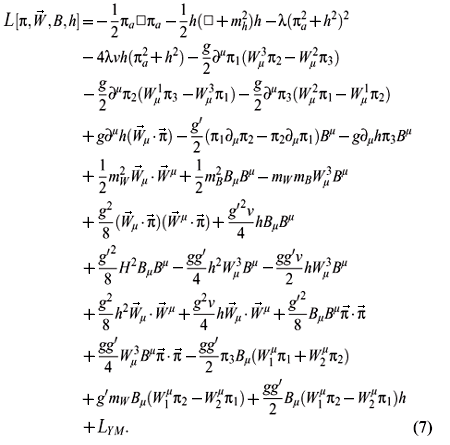Thus we have a gauge theory spontaneously broken with the= (p1,p2,p3) fields as the would-be Goldstone bosons of the broken symmetry SU(2)L Ä U(1)Y/U(1)Q and pa fields as the would-be Goldstone bosons of the broken global symmetry O(N + 1)/O(N).

The theory for the large N limit makes sense when N ® ¥ and gives rise to finite amplitudes for different processes. To get finite amplitudes is necessary to choose appropriate parameters in the large N limit. We will take the following definitionin order to use perturbative expansion of the strongly interacting sector as a function of the l parameter. With this definition, physical masses must be finite and independent of N in the large N limit. From the masseswe obtain for the other parameters of the model in the large N limit the following valuesFinally, we obtain the Feynman rules necessary to calculate the decay widths for h ® W+W- and h ® p+p- in the large N limit, see Fig. 1.

III. THE HIGGS BOSON DECAY AND THE EQUIVALENCE THEOREM

The SM in the large N limit is associated with the O(N + 1)/O(N) and SU(2)L Ä U(1)Y/U(1)Q global and local symmetry breaking schemes respectively. We can calculate the amplitudes for h ® W+W- and h ® p+p- decays in order to show that the ET holds in the proposed scenario.

Feynman diagrams at tree level in this approximation are of the order of O(g) or O(g') and of the order of O(1/) in the large N limit . The decay widths at tree level for h ® W+W- and h ® p+p- processes are given byTo obtain the amplitudes at next-to-leading order is necessary to introduce the radiative corrections. First we calculate the self-energy of the scalar particle h, whose Feynman diagrams at next-to-leading order are shown in Fig. 2. In this case, the self-energy at one loop level with pa fields into the loops is of the order of 1/N times N where N is the number of degrees of freedom running into the loop. Therefore, radiative corrections are of the order of one in the large N limit. The same analysis can be done for the self-energy diagram with l loops. It has two vertices with hpp and l - 1 vertices with four pa and is of the order of (1/)21/N)l-1 times Nl the number of pion fields running into the l loops. Consequently, the self-energy diagram with l loops is of the order of one in the large N limit. However, the one irreducible particle function (1IP) for self-energy diagram with, Zm into the loop is of the order of 1/N, which is negligible in the large N limit.

After doing all calculations by using dimensional regularization, with d = 4 - e integrals from the loops, we find (see appendixappendix)with Iq given bywhere D = 2/e + log4p - ge and µ is the renormalization scale. The choice of the renormalization scale µ is arbitrary. Therefore, we shall adopt µ 1 TeV as a reasonable choice. We have taken into account that only the one irreducible particle functions are important in perturbation theory for the renormalization of parameters such as the mass and the wave function .

From the self-energy calculation the wave function renormalization of the Higgs boson can be obtained asThe contributions to p±, W± and Zm self-energies are proportional to 1/N and in the large N limit we obtain that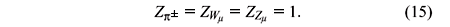To calculate the Higgs decays at this order, vertex corrections have to be included as well, as shown in Fig. 3.

The radiative corrections of hW+W- vertex displayed in Fig. 3(a) are suppressed since they are of the order of 1/N2 becoming negligible in our approximation. The hW+W- vertex can be written at this level as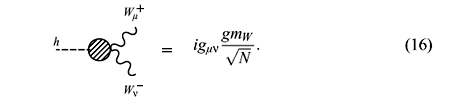For the hp+p- vertex corrections shown in Fig. 3(b), the pions into the loops give the most important contributions and can be written as

where the W± contributions into the loops are suppressed by a 1/N factor with respect to the pa contributions. Similarly, the contribution with a higgs running into the loop is also suppressed by a 1/N factor.

The wave function renormalization of the Higgs particle Eq.(14) and the vertex radiative correction Eq. (3) diverge. To obtain finite amplitudes the Higgs mass has to be renormalized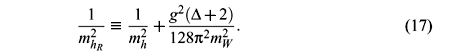The real part of the Zh function Eq.(14) is given by

In the same way, the real part of the vertex correction Eq.(3) can be expressed as

Hence, the vertex corrections Eq.(19) multiplied by the factors of the renormalized wave functions

Zp give rise to the h ® p+p- amplitude at next-to-leading order and can be written at O(g2/) as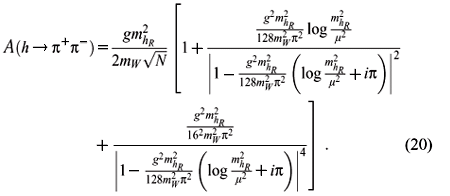The same procedure is done for the h ® W+W- amplitude where the vertex corrections are multiplied by the factors of the renormalized wave functions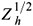ZW, and can be written at O(g2/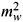) asIn order to show that the ET holds for non-perturbative next-to-leading order, we calculate Higgs decays into gauge bosons and pions in the large N limit. We then compare the decay widths as obtained from the decay amplitudes for h ® p+p- and h ® W+W- in Eqs. (20) and (21) respectively. Such decay widths are given byandIn Fig. 4 we display the ratio G(h ® p+p-) /G(h ® W+W-) as a function of the Higgs mass including next-to-leading order corrections. From this figure it can be seen that such quotient tends to one for large Higgs masses (4.5 TeV), showing the validity of the ET at high energies.

IV. UNITARY IN THE LARGE N LIMIT

As a consequence of unitarity of the S-matrix, i. e. SS = 1, the Optical Theorem is obtained . By defining S = 1 + iT, where the T is called the transition matrix, we haveand since four momentum is conserved in the transition from initial state |iñ to final state |fñ, we can always writeandInserting a complete set of intermediate states |qñ we findand from the identity (24) we can obtain the Cutkosky's rulewhere the sum runs over all possible sets of intermediate states qi.

Applying this identity to the decay G(h ® p+p-) we findIn the left-hand side of the previous equation we have the imaginary part of the product of Eq. (3) times the wave function

Zp, resulting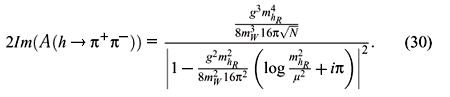For the right-hand side, we have to multiply the amplitudes calculated in the large N limit

i.e.,by

i.e.,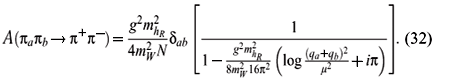Then the right-hand side of Eq. (29) becomeswhere 1/4 is the symmetry factor for identical bosons in the final state.

From Eq. (29) we defineand the integral over qb can be written asIntegrating the four-dimensional delta function in Eq.(36) we obtainIn the center-of-mass framethe integral in Eq. (36) can be rewritten asand by using p2 =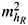Comparing equations (30) and (39) we see that the Higgs decay G(h ® p+p-) calculated in the large N limit at next-to-leading order fulfills the unitarity condition.

V. CONCLUSIONS

We have shown that non-perturbative calculations at next-to-leading order in the large N limit for the case of a Higgs decaying into W± and p± fulfill the ET. In particular, we found that the decay widths G(h ® W+W-) and G(h ® p+p-) get values that are basically identical for heavy Higgs bosons i.e.4.5 TeV.

On the other hand, we have also shown that calculations in the same scheme for the Higgs decaying into pions respect unitarity. This results open the possibility to study strongly interacting systems as could be the case of the SM with a heavy Higgs boson.

Acknowledgments

We thank to Alexis Rodriguez and Marek Nowakowski for reading the manuscript and their comments. We also thank COLCIENCIAS, DIB, and DINAIN for their financial support.

REFERENCES

 S. Weinberg, Phys. Lett. 19, 1264 (1967); S.L. Glashow, Nucl. Phys. B 20, 579 (1961).

 Particle Data Group, Phys. Rev. D 66, 1 (2002).

 LEP Electroweak Working Group (2004), http://lepewwg.web.cern.ch/LEPEWWG/.

 R. S. Chivukula and N. Evans, Phys. Lett. B 464, 244 (1999); R. S. Chivukula, N. Evans, and C. Hoelbling. Phys. Rev. Lett. 85, 511 (2000); J. Bagger, A. Falk, and M. Schwartz, Phys. Rev. Lett. 84, 1385 (2000); Michael E. Peskin, Phys. Rev. D 64, 093003 (2001).

 B. Lee, C. Quigg, and H. Thacker, Phys. Rev. D 16, 1519 (1977); W. Marciano, G. Valencia, and S. Willenbrock, Phys. Rev. D 40, 1725 (1989).

 N. Cabibbo, L. Maiani, G. Parisi, and R. Petronzio, Nucl. Phys. B 158, 295 (1979).

 M. Sher, Phys. Rep. 179, 273 (1989); M. Quiros, Perspectives on Higgs Physics II, Ed. G.L. Kane, World Scientific, Singapore [arXiv: hep-ph/9703412].

 Christopher Kolda, Hitoshi Murayama, JHEP 0007, 035 (2000).

 D.J.E Callaway, Phys. Rep. 167, 241 (1988); T. Hambye and K. Riesselmann, Phys. Rev. D 55, 7255 (1997); J.S. Lee and J.K. Kim, Phys. Rev. D 53, 6689 (1996).

 A. Dobado, J. Morales, J.R. Pelaez, and M.T. Urdiales, Phys. Lett. B 387, 563 (1996); A. Ghinculov, T. Binoth, and J.J. van der Bij, Phys. Rev. D 57, 1487 (1998).

 A. Ghinculov, Nucl. Phys. B 455, 21 (1995); A. Frink et. al., Phys. Rev. D 54, 4548 (1996).

 P. Maher, L. Durand, and K. Riesselmann, Phys. Rev. D 48, 1061 (1993); 52, 553 (1995); K. Riesselmann, Phys. Rev. D 53, 6626 (1996).

 U. Nierste and K. Riesselmann, Phys. Rev. D 53, 6638 (1996).

 W.J. Marciano and S.S.D. Willenbrock Phys. Rev. D 37, 2509 (1988).

 B.W. Lee, C. Quigg, and H. Thacker Phys. Rev. D 16, 1519 (1977). J.M. Cornwall, D.N. Levin, and G. Tiktopoulus, Phys. Rev. D 10, 1145 (1974). M.S. Chanowitz and M.K. Gallaird Nucl. Phys. B 261, 379 (1985). G.K. Gounaris, R. Kogerler, and H. Neufeld, Phys. Rev. D 34, 3257 (1986). H.J. He and W. Kilgore, Phys. Rev. D 55, 1515 (1997).

 A. Dobado and M. Herrero, Phys. Lett. B 228, 495 (1989). J. Donoghue and C. Ramirez, Phys. Lett. B 234, 361 (1990).

 J. Gasser and H. Leutwyler, Ann. of Phys. 158, 142 (1984). S. Weinberg, Phys. Rev. Lett. 19, 1264 (1967); M.S. Chanowitz, M. Golden, and H. Georgi, Phys. Rev. D 36, 1490 (1987); A. Longhitano, Phys. Rev. D 22, 1166 (1980); Nucl. Phys. B 188, 118 (1981).

 H. Veltman, Phys. Rev. D 41, 264 (1990).

 A. Dobado, J.R. Pelaez, and M. T. Urdiales, Phys. Rev. D 56, 7133 (1997).

 A. Dobado, A. Lopez, and J. Morales, Il Nuovo Cimento A 108, 335 (1995); A. Dobado and J. Morales, Phys. Rev. D 52, (1995) 2878.

 S. Coleman, R. Jackiw, and H. D. Politzer, Phys. Rev. D 10 2491 (1974); Dolan and Jackiw, Phys. Rev. D 9, 3320 (1974); M. Kobayashi and T. Kugo, Prog. Theor. Phys. 54, 1537 (1975); W. Bardeen and M. Moshe, Phys. Rev. D 28, 1372 (1983).

 R.Casalbuoni, D. Dominici, and R. Gatto, Phys. Lett. B 147, 419 (1984); M.B. Einhorn, Nucl. Phys. B 246, 75 (1984); A. Dobado and J.R. Pelaez, Phys. Lett. B 286, 136 (1992).

 H. Georgi, Lie Algebras in Particle Physics, Addison Wesley, Frontiers in Physics. New York 1982.

 M. E. Peskin, D. V. Schroeder, An introduction to quantum field theory, Westview Press (1995), Chap. 7.

 R. E. Cutkosky, J. Math. Phys. 1, 429 (1960).

In this appendix we show the explicit calculation of a Feynman diagram with l loops in the large N limit that contributes to the Higgs boson self-energy.where 1/2l is the symmetry factor of the diagram. The first factor corresponds to the initial and final loops times the vertices with three particles, the second factor represents the product of the l - 1 internal vertices with four interacting fields and the last factor correspond to l - 2 loops. Each loop contributes with an N factor, as they have N circulating pions.

•  S. Weinberg, Phys. Lett. 19, 1264 (1967);
• S.L. Glashow, Nucl. Phys. B 20, 579 (1961).
•  Particle Data Group, Phys. Rev. D 66, 1 (2002).
•  R. S. Chivukula and N. Evans, Phys. Lett. B 464, 244 (1999);
• R. S. Chivukula, N. Evans, and C. Hoelbling. Phys. Rev. Lett. 85, 511 (2000);
• J. Bagger, A. Falk, and M. Schwartz, Phys. Rev. Lett. 84, 1385 (2000);
• Michael E. Peskin, Phys. Rev. D 64, 093003 (2001).
•  B. Lee, C. Quigg, and H. Thacker, Phys. Rev. D 16, 1519 (1977);
• W. Marciano, G. Valencia, and S. Willenbrock, Phys. Rev. D 40, 1725 (1989).
•  N. Cabibbo, L. Maiani, G. Parisi, and R. Petronzio, Nucl. Phys. B 158, 295 (1979).
•  M. Sher, Phys. Rep. 179, 273 (1989);
• M. Quiros, Perspectives on Higgs Physics II, Ed. G.L. Kane, World Scientific, Singapore [arXiv: hep-ph/9703412].
•  Christopher Kolda, Hitoshi Murayama, JHEP 0007, 035 (2000).
•  D.J.E Callaway, Phys. Rep. 167, 241 (1988);
• T. Hambye and K. Riesselmann, Phys. Rev. D 55, 7255 (1997);
• J.S. Lee and J.K. Kim, Phys. Rev. D 53, 6689 (1996).
•  A. Dobado, J. Morales, J.R. Pelaez, and M.T. Urdiales, Phys. Lett. B 387, 563 (1996);
• A. Ghinculov, T. Binoth, and J.J. van der Bij, Phys. Rev. D 57, 1487 (1998).
•  A. Ghinculov, Nucl. Phys. B 455, 21 (1995);
• A. Frink et. al., Phys. Rev. D 54, 4548 (1996).
•  P. Maher, L. Durand, and K. Riesselmann, Phys. Rev. D 48, 1061 (1993); 52, 553 (1995);
• K. Riesselmann, Phys. Rev. D 53, 6626 (1996).
•  U. Nierste and K. Riesselmann, Phys. Rev. D 53, 6638 (1996).
•  W.J. Marciano and S.S.D. Willenbrock Phys. Rev. D 37, 2509 (1988).
•  B.W. Lee, C. Quigg, and H. Thacker Phys. Rev. D 16, 1519 (1977).
• J.M. Cornwall, D.N. Levin, and G. Tiktopoulus, Phys. Rev. D 10, 1145 (1974).
• M.S. Chanowitz and M.K. Gallaird Nucl. Phys. B 261, 379 (1985).
• G.K. Gounaris, R. Kogerler, and H. Neufeld, Phys. Rev. D 34, 3257 (1986).
• H.J. He and W. Kilgore, Phys. Rev. D 55, 1515 (1997).
•  A. Dobado and M. Herrero, Phys. Lett. B 228, 495 (1989).
• J. Donoghue and C. Ramirez, Phys. Lett. B 234, 361 (1990).
•  J. Gasser and H. Leutwyler, Ann. of Phys. 158, 142 (1984).
• S. Weinberg, Phys. Rev. Lett. 19, 1264 (1967);
• M.S. Chanowitz, M. Golden, and H. Georgi, Phys. Rev. D 36, 1490 (1987);
• A. Longhitano, Phys. Rev. D 22, 1166 (1980); Nucl. Phys. B 188, 118 (1981).
•  H. Veltman, Phys. Rev. D 41, 264 (1990).
•  A. Dobado, J.R. Pelaez, and M. T. Urdiales, Phys. Rev. D 56, 7133 (1997).
•  A. Dobado, A. Lopez, and J. Morales, Il Nuovo Cimento A 108, 335 (1995);
• A. Dobado and J. Morales, Phys. Rev. D 52, (1995) 2878.
•  S. Coleman, R. Jackiw, and H. D. Politzer, Phys. Rev. D 10 2491 (1974);
• Dolan and Jackiw, Phys. Rev. D 9, 3320 (1974);
• M. Kobayashi and T. Kugo, Prog. Theor. Phys. 54, 1537 (1975);
• W. Bardeen and M. Moshe, Phys. Rev. D 28, 1372 (1983).
•  R.Casalbuoni, D. Dominici, and R. Gatto, Phys. Lett. B 147, 419 (1984);
• M.B. Einhorn, Nucl. Phys. B 246, 75 (1984);
• A. Dobado and J.R. Pelaez, Phys. Lett. B 286, 136 (1992).
•  H. Georgi, Lie Algebras in Particle Physics, Addison Wesley, Frontiers in Physics New York 1982.
•  M. E. Peskin, D. V. Schroeder, An introduction to quantum field theory, Westview Press (1995), Chap. 7.
•  R. E. Cutkosky, J. Math. Phys. 1, 429 (1960).

# Publication Dates

• Publication in this collection
13 Oct 2005
• Date of issue
Sept 2005